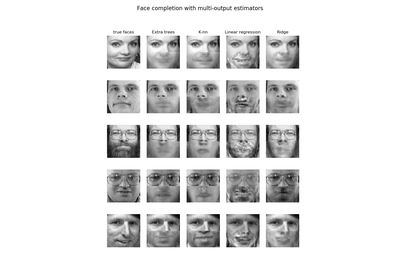# 3.2.4.1.9. `sklearn.linear_model`.RidgeCV¶

class `sklearn.linear_model.``RidgeCV`(alphas=(0.1, 1.0, 10.0), fit_intercept=True, normalize=False, scoring=None, cv=None, gcv_mode=None, store_cv_values=False)[source]

Ridge regression with built-in cross-validation.

By default, it performs Generalized Cross-Validation, which is a form of efficient Leave-One-Out cross-validation.

Read more in the User Guide.

Parameters: alphas : numpy array of shape [n_alphas] Array of alpha values to try. Regularization strength; must be a positive float. Regularization improves the conditioning of the problem and reduces the variance of the estimates. Larger values specify stronger regularization. Alpha corresponds to `C^-1` in other linear models such as LogisticRegression or LinearSVC. fit_intercept : boolean Whether to calculate the intercept for this model. If set to false, no intercept will be used in calculations (e.g. data is expected to be already centered). normalize : boolean, optional, default False This parameter is ignored when `fit_intercept` is set to False. If True, the regressors X will be normalized before regression by subtracting the mean and dividing by the l2-norm. If you wish to standardize, please use `sklearn.preprocessing.StandardScaler` before calling `fit` on an estimator with `normalize=False`. scoring : string, callable or None, optional, default: None A string (see model evaluation documentation) or a scorer callable object / function with signature `scorer(estimator, X, y)`. cv : int, cross-validation generator or an iterable, optional Determines the cross-validation splitting strategy. Possible inputs for cv are: None, to use the efficient Leave-One-Out cross-validation integer, to specify the number of folds. An object to be used as a cross-validation generator. An iterable yielding train/test splits. For integer/None inputs, if `y` is binary or multiclass, `sklearn.model_selection.StratifiedKFold` is used, else, `sklearn.model_selection.KFold` is used. Refer User Guide for the various cross-validation strategies that can be used here. gcv_mode : {None, ‘auto’, ‘svd’, eigen’}, optional Flag indicating which strategy to use when performing Generalized Cross-Validation. Options are: ```'auto' : use svd if n_samples > n_features or when X is a sparse matrix, otherwise use eigen 'svd' : force computation via singular value decomposition of X (does not work for sparse matrices) 'eigen' : force computation via eigendecomposition of X^T X ``` The ‘auto’ mode is the default and is intended to pick the cheaper option of the two depending upon the shape and format of the training data. store_cv_values : boolean, default=False Flag indicating if the cross-validation values corresponding to each alpha should be stored in the cv_values_ attribute (see below). This flag is only compatible with cv=None (i.e. using Generalized Cross-Validation). cv_values_ : array, shape = [n_samples, n_alphas] or shape = [n_samples, n_targets, n_alphas], optional Cross-validation values for each alpha (if store_cv_values=True and cv=None). After fit() has been called, this attribute will contain the mean squared errors (by default) or the values of the {loss,score}_func function (if provided in the constructor). coef_ : array, shape = [n_features] or [n_targets, n_features] Weight vector(s). intercept_ : float | array, shape = (n_targets,) Independent term in decision function. Set to 0.0 if `fit_intercept = False`. alpha_ : float Estimated regularization parameter.

`Ridge`
Ridge regression
`RidgeClassifier`
Ridge classifier
`RidgeClassifierCV`
Ridge classifier with built-in cross validation

Methods

 `fit`(X, y[, sample_weight]) Fit Ridge regression model `get_params`([deep]) Get parameters for this estimator. `predict`(X) Predict using the linear model `score`(X, y[, sample_weight]) Returns the coefficient of determination R^2 of the prediction. `set_params`(**params) Set the parameters of this estimator.
`__init__`(alphas=(0.1, 1.0, 10.0), fit_intercept=True, normalize=False, scoring=None, cv=None, gcv_mode=None, store_cv_values=False)[source]
`fit`(X, y, sample_weight=None)[source]

Fit Ridge regression model

Parameters: X : array-like, shape = [n_samples, n_features] Training data y : array-like, shape = [n_samples] or [n_samples, n_targets] Target values. Will be cast to X’s dtype if necessary sample_weight : float or array-like of shape [n_samples] Sample weight self : Returns self.
`get_params`(deep=True)[source]

Get parameters for this estimator.

Parameters: deep : boolean, optional If True, will return the parameters for this estimator and contained subobjects that are estimators. params : mapping of string to any Parameter names mapped to their values.
`predict`(X)[source]

Predict using the linear model

Parameters: X : {array-like, sparse matrix}, shape = (n_samples, n_features) Samples. C : array, shape = (n_samples,) Returns predicted values.
`score`(X, y, sample_weight=None)[source]

Returns the coefficient of determination R^2 of the prediction.

The coefficient R^2 is defined as (1 - u/v), where u is the residual sum of squares ((y_true - y_pred) ** 2).sum() and v is the total sum of squares ((y_true - y_true.mean()) ** 2).sum(). The best possible score is 1.0 and it can be negative (because the model can be arbitrarily worse). A constant model that always predicts the expected value of y, disregarding the input features, would get a R^2 score of 0.0.

Parameters: X : array-like, shape = (n_samples, n_features) Test samples. y : array-like, shape = (n_samples) or (n_samples, n_outputs) True values for X. sample_weight : array-like, shape = [n_samples], optional Sample weights. score : float R^2 of self.predict(X) wrt. y.
`set_params`(**params)[source]

Set the parameters of this estimator.

The method works on simple estimators as well as on nested objects (such as pipelines). The latter have parameters of the form `<component>__<parameter>` so that it’s possible to update each component of a nested object.

Returns: self :

## 3.2.4.1.9.1. Examples using `sklearn.linear_model.RidgeCV`¶Face completion with a multi-output estimators